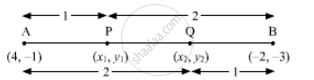# Find the Coordinates of the Points of Trisection of the Line Segment Joining (4, -1) and (-2, -3). - Mathematics

Find the coordinates of the points of trisection of the line segment joining (4, -1) and (-2, -3).

#### SolutionLet P (x1y1) and Q (x2y2) are the points of trisection of the line segment joining the given points i.e., AP = PQ = QB

Therefore, point P divides AB internally in the ratio 1:2.

x_1= (1xx(-2)+2xx4)/(1+2), y_1 = (1xx(-3)+2xx(-1))/(1+2)

x_1 = (-2+8)/3=6/3=2, y_1 = (-3-2)/3 = (-5)/3

Therefore P(x1,y1) = (2, -5/3)

Point Q divides AB internally in the ratio 2:1.

x_2=(2xx(-2)+1xx4)/(2+1), y_2=(2xx(-3)+1xx(-1))/(2+1)

x_2 = (-4+4)/3 = 0, y_2= (-6-1)/3 = (-7)/3

Q(x_2, y_2) = (0, -7/3)

Concept: Section Formula
Is there an error in this question or solution?
Chapter 7: Coordinate Geometry - Exercise 7.2 [Page 167]

#### APPEARS IN

NCERT Class 10 Maths
Chapter 7 Coordinate Geometry
Exercise 7.2 | Q 2 | Page 167

Share